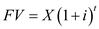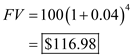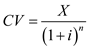# Quiz 17: Financial Economics

The future value ( FV ) of the investment is calculated using the formula of future value model. The formula for this is given as:Where "X " is the amount of money today " i " is the interest rate. " t " is the duration or the number of years. On substitution:Thus, future value of the investment is \$116. 985.
The current value ( CV ) of the investment is calculated using the formula of present value model. The formula for this is given as:Where "X" is the amount of money received in the future " i " is the interest rate. " n " is the duration or the number of years. On substitution:Thus, the current value of the investment is \$82,644.62.